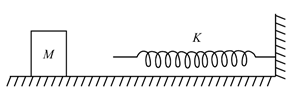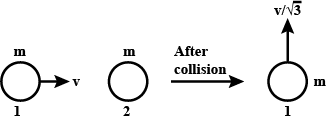# Work Power and Energy Quiz with Answers

This online quiz contains 20 work, energy and power test questions for class 11 physics chapter 6. This mcq online practice problems with answers also has assertion and reason type questions. To evaluate your performance of this chapter try and solve these test questions in stipulated amount of time. This is not a timed test so keep track of how much time you are taking in solving this test. Answers to all the questions along with solutions can be found after submitting this test by clicking ‘submit’ button.

## Multiple Choice Questions on Work, Energy and Power

General Instructions

1. Your test contains multiple-choice questions with only one answer type of questions. There are a total of 20 questions
2. This is a 45 min test. Please make sure you complete it in the stipulated time
3. You can finish this test any time using the 'Submit' button.
1. If a machine is lubricated with oil
2. An ideal spring with spring constant k is hung from the ceiling and a block of mass M is attached to its lower end. The mass is released with the spring initially unstretched. Then the maximum extension in the spring is
3. A particle is acted by a force $F=kx$, where $k$ is a positive constant. Its potential energy at $x=0$ is zero. Which curve correctly represents the variation of the potential energy of the block with respect to $x$?

4. A force $\vec{F}=\left(5\hat{i}+3\hat{j}+2\hat{k}\right)N$ is applied over a particle which displaces it from its origin to the point $\vec{r}=\left(2\hat{i}-\hat{j}\right)m$. The work done on the particle (in joule) is
5. A particle of mass 100 g is thrown vertically upwards with a speed of 5 m/s. The work done by the force of gravity during the time the particle goes up is
6. A ball whose Kinetic Energy is $E$, is projected at an angle of ${45}^0$ to the horizontal. The kinetic energy of the ball at the highest point of its flight will be

7. The potential energy of a one Kg particle free to move along the x-axis is given by
$V\left(x\right)=\frac{x^4}{4}-\frac{x^2}{2}$ (in Joule)
The total mechanical energy of the particle is $2J$. Then maximum speed (in m/s) is
8. The block of mass $M$ moving on the frictionless horizontal surface collides with the spring of spring constant $k$ and compress it by length $L$.The maximum momentum of the block after the collision is
9. A bomb of mass 16 Kg at rest explodes into two pieces of masses 4Kg and 12 Kg. The velocity of the 12 Kg mass is 4 m/s. The kinetic energy of the other mass is
10. A mass m moves with velocity v and collides elastically with another identical mass at rest. After the collision, the first mass moves with velocity v/\sqrt3 in a direction perpendicular to the initial direction of motion.Find the speed of the second mass after the collision.
11. Statement 1: Two particles moving in the same direction do not lose all their energy in a completely inelastic collision.

Statement 2: Principle of conservation of momentum holds true for all kinds of collision.
12. A block is kept on an inclined plane of inclination $\theta$ and length $l$. The velocity of the particle at bottom of the incline (the coefficient of friction is $\mu$ ) is
13. A bullet hits and gets embedded in a solid block resting on a frictionless surface. In this process which one of the following is correct?
14. If a spring extends by $x$ on loading, then the energy stored by the spring is (if $T$ is tension in the spring and $K$ is spring constant)
15. Which of the following is true
16. Two particles are seen to collide and move jointly together after the collision, for the total system,
17. A ball is bouncing down the flight of stairs. The coefficient of restitution is $e$. The height of each step is d and the ball descends one step each bounce. After each bounce, it rebounds to a height $h$ above the next lower step. The height is large enough compared with the width of step so that the impacts are effectively head-on. Find the relationship between $h$ and $d. 18. In the following question, a statement of assertion is followed by a statement of reason. Assertion: When a body moves along a circular path, no work is done by the centripetal force. Reason: The centripetal force is used in moving the body along the circular path and hence no work is done. Mark the correct choice as 19. In the following question, a statement of assertion is followed by a statement of reason. Assertion: Mass and energy are not conserved separately, but are conserved as single entity called mass-energy. Reason: Mass and energy are inter-convertible in accordance with Einstein’s relation,$E=mc^2\$

Mark the correct choice as
20. In the following question, a statement of assertion is followed by a statement of reason.

Assertion: In elastic collision of two billiard balls, the total kinetic energy is conserved during the short time of collision of the balls (i.e., when they are in contact)

Reason: Energy spent against friction does not follow the law of conservation of energy.
Mark the correct choice as
21. In the following question, a statement of assertion is followed by a statement of reason.

Assertion: If the momentum of a body is increased by 50%, its kinetic energy will increase by 125%

Reason: Kinetic energy is proportional to square of velocity.
Mark the correct choice as

Subscribe
Notify ofThis site uses Akismet to reduce spam. Learn how your comment data is processed.

Inline Feedbacksyash
2 years ago

NiceGhaffar Ali
1 year ago

PhysicaRohit
1 year ago

I ned more practiceM. Hruday
1 month ago

For 12th question sol. Correct but option is wrong

5
0